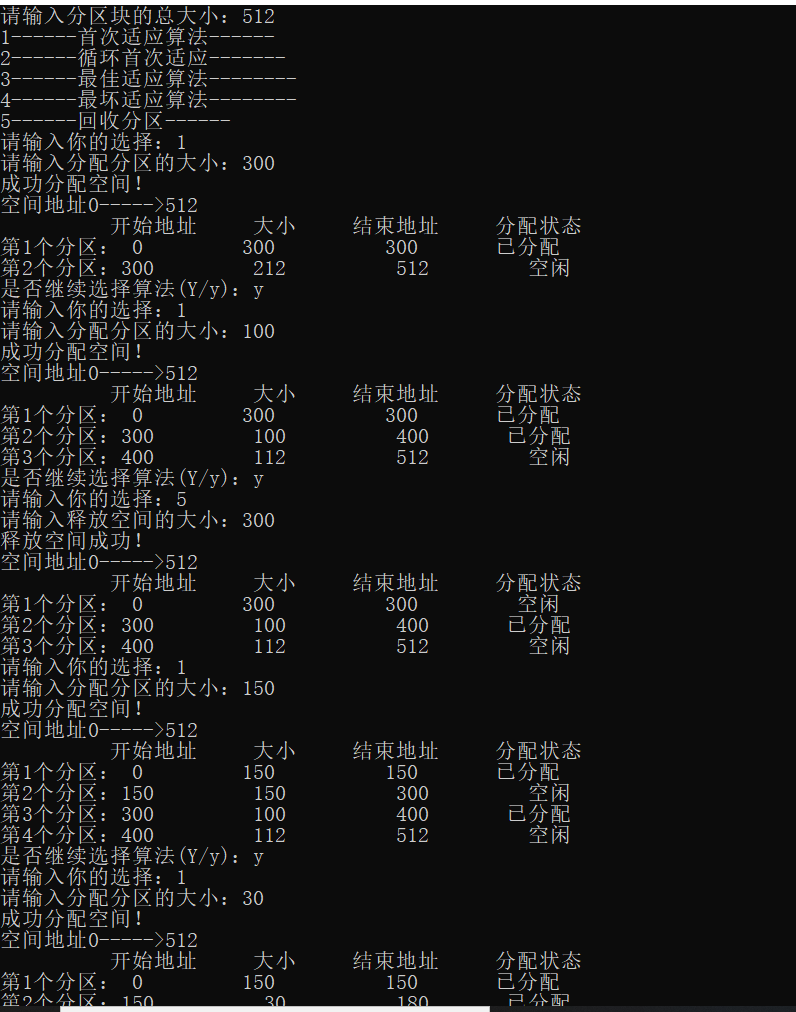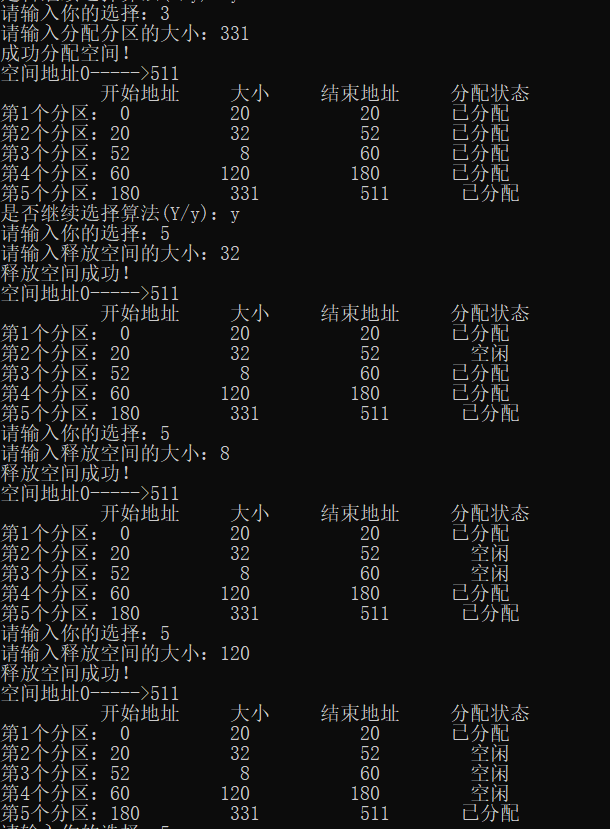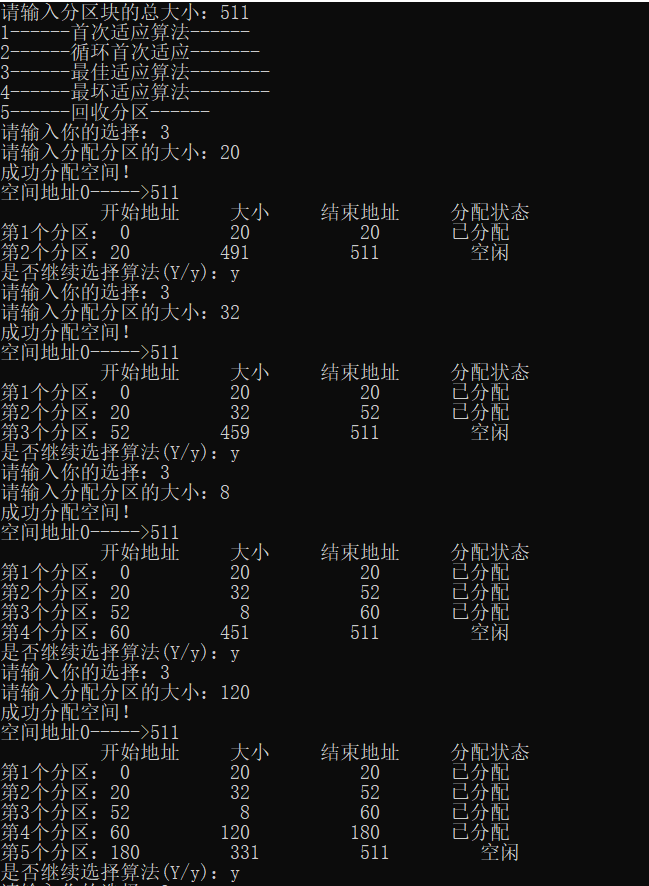• 基于C++实现内存动态分区分配算法
千次阅读
2019-12-26 14:39:23

数据结构使用的是结构体 + 链表的方式来实现的
源代码在下方github网址中
github网站
构思过程

更多相关内容
• 动态分区分配算法,完整，经严谨测试 操作系统实验必备
• 用C/C++实现一个完整的（可变）动态分区管理器，包括分配，回收，分区碎片整理等。希望同学们实现如下功能： 初始化功能：内存状态设置为初始状态。 分配功能：要求至少使用两种算法，用户可以选择使用。 回收...
• 任务要求 了解动态分区分配中使用的数据结构和分配算法，并进一步加深对动态分区存 储管理方式及其实现过程的理解。 任满杰等 《操作系统原理实用教程》 电子工业出版社 2006 汤子瀛 《计算机操作系统》（修订版）...

课 程 设 计

课程设计名称： 操作系统课程设计

专 业 班 级 ：

学 生 姓 名 ：

学 号 ：

指 导 教 师 ：

课程设计时间： 6月13 日-——6月17 日

1

计算机科学 专业课程设计任务书

学生姓名 马飞扬 专业班级 学号

题 目 动态分区分配方式的模拟 1

课题性质 其它 课题来源 自拟课题

指导教师 同组姓名

1)用C语言实现采用首次适应算法的动态分区分配过程alloc()和回收过程

free()。其中，空闲分区通过空闲分区链表来管理，在进行内存分配时，系统

优先使用空闲区低端的空间。

2)假设初始状态如下，可用的内存空间为640KB，并有下列的请求序列；

主要内容 作业 1申请 130KB；作业2 申请60KB；作业3 申请 100KB；作业2释放

60KB；作业4 申请200 KB；作业3释放 100 KB；作业 1释放 130 KB；

作业5 申请 140 KB；作业6 申请60 KB；作业7 申请50KB；作业6释放

60 KB

请采用首次适应算法进行内存块的分配和回收，同时显示内存块分配和回收后

空闲内存分区链的情况。

任务要求 了解动态分区分配中使用的数据结构和分配算法，并进一步加深对动态分区存

储管理方式及其实现过程的理解。

任满杰等 《操作系统原理实用教程》 电子工业出版社 2006

汤子瀛 《计算机操作系统》(修订版)西安电子科技大学出版社 2001

张尧学 史美林 《计算机操作系统教程》实验指导 清华大学出版社 2000

罗宇等 《操作系统课程设计》机械工业出版社 2005

参考文献

2

指导教师签字：

审查意见

教研室主任签字： 年 月 日

说明：本表由指导教师填写，由教研室主任审核后下达给选题学生，装订在设计 (论文)首页

1：需求分析

(1)用C语言实现采用首次适应算法的动态分区分配过程alloc()和回收过程

free()。其中，空闲分区通过空闲分区链表来管理，在进行内存分配时，

系统优先使用空闲区低端的空间。

(2)假设初始状态下，可用的内存空间为640KB，并有下列的请求序列：作

业 1申请 130KB；作业2 申请60KB；作业3 申请 100KB；作业2释

放60KB；作业4 申请200KB；作业3释放 100KB；作业 1释放 130

KB；作业5申请 140 KB；作业6 申请60 KB；作业7 申请50KB；作

业6释放60KB。采用首次适应算法进行内存块的分配和回收，同时显示

内存块分配和回收后空闲内存分区链的情况。

2 ：概要设计

1

() 数据结构：作业队列数据结构，用于存储待处理作业；阻塞作业队列数据

结构，用于存储阻塞的作业。已分配内存块的双向链表，记录当前系统已

分配的各个内存块；未分配内存块的双向链表，记录系统中剩余的各个内

存块；系统内存分配总情况的结点对象，记录系统中阻塞的作业总数，已

分配的内存块数，剩余的内存块数。

2

() 主函数：对作业队列、阻塞队列、已分配内存块链表

展开全文• 编写一个动态分区分配算法模拟程序，加深对动态分区存储管理方式及其实现过程的理解。 要求： 1.空闲分区通过空闲区链进行管理，在内存分配时，优先考虑低地址部分的空闲区。 2.分别采用首次适应算法、最佳适应算法...
• #include <iostream> using namespace std; const int MaxNumber = 100; int FreePartition[MaxNumber];...//需要分配的进程个数 //首次适应算法 void FF() { //寻找大小能满足进程要求的分区 for
#include <iostream>
using namespace std;
const int MaxNumber = 100;
int FreePartition[MaxNumber];//空闲可用分区
int Partition[MaxNumber];//进程所需分区
int  PartitionNum, ProcessNum;
int n; //空闲分区个数
int m;//需要分配的进程个数
//首次适应算法
void FF() {
//寻找大小能满足进程要求的分区
for (int i = 0; i < m; i++) {
for (int j = 0; j < n; j++) {
if (Partition[i] <= FreePartition[j]) {
FreePartition[j]=0;
cout << "第 " << i+1 << "个进程被分配给了分区 " << j << endl; break;
}
if (j == n - 1) {
cout << "系统没有足够大的内存分配给进程 " << i << " ，内存分配失败！" << endl;
}
}
}
}
//循环首次适应算法
void NF() {
int lastUsed = 0;
int count = 0;
bool check;
while (count < m) {
if (lastUsed == n) {
lastUsed -= n;
}
for (int i = lastUsed; i < (n + lastUsed); i++) {
if (i < n) {
if (FreePartition[i] >= Partition[count]) {
FreePartition[i] =0;
cout << "空闲分区" << i << "分配了" << Partition[count] << "给进程" << count << endl;
lastUsed = i+1;
break;
}
}
else {
if (FreePartition[i - n] >= Partition[count]) {
FreePartition[i - n]=0;
cout << "空闲分区" << i - n << "分配了" << Partition[count] << "给进程" << count << endl;
lastUsed = i+1; break;
}
}
//没有足够大的进程
if (i == n + lastUsed - 1) {
cout << "系统没有足够大的内存分配给进程" << count << "，内存分配失败！" << endl;
}
}
count++;
}
}
//最佳适应算法
void BF() {
int count = 0;
int *Number = new int[n];//记录每个分区内存和进程的内存差
//让内存最小的进程先判断
int *Temp = new int[m];
for (int i = 0; i < m; i++)
{
Temp[i] = Partition[i];
}
int *number = new int[m];
for (int i = 0; i < m; i++)
{
number[i] = i;
}
for (int i = 0; i < m; i++)
{
for (int j = i + 1; j < m; j++)
{
if (Temp[i] > Temp[j]) {
int num = 0;
num = Temp[i];
Temp[i] = Temp[j];
Temp[j] = num;

num = number[i];
number[i] = number[j];
number[j] = num;
}
}
}

while (count < m) {
//为进程分配内存
for (int i = 0; i < n; i++) {
Number[i] = (FreePartition[i] - Partition[number[count]]) >= 0 ? (FreePartition[i] - Partition[number[count]]) : 10000;//如果分区内存大于进程所需，设为10000表示无效分区
}
int a = 0;//记录最合适分区的位置
for (int i = 1; i < n; i++)
{
//只需要求数组第一个，即相差最小的
if (Number[i] < Number) {
int num = 0;
a = i;
num = Number[i];
Number[i] = Number;
Number = num;
}

}
if (FreePartition[a] >= Partition[number[count]]) {
FreePartition[a] = 0;
cout << "分区" << a << "分配给了进程" << number[count] << endl;
}
else {
cout << "系统没有足够大的内存分配给进程 " << number[count] << " ，内存分配失败！" << endl;
}
count++;
}
}
//最坏适应算法
void WF() {
int count = 0;
int *Number = new int[n];//记录每个分区内存和进程的内存差
//让内存最大的进程先判断
int *Temp = new int[m];
for (int i = 0; i < m; i++)
{
Temp[i] = Partition[i];
}
int *number = new int[m];
for (int i = 0; i < m; i++)
{
number[i] = i;
}
for (int i = 0; i < m; i++)
{
for (int j = i+1; j < m; j++)
{
if (Temp[i] < Temp[j]) {
int num = 0;
num = Temp[i];
Temp[i] = Temp[j];
Temp[j] = num;

num = number[i];
number[i] = number[j];
number[j] = num;
}
}
}

while (count < m) {
//为进程分配内存
for (int i = 0; i < n; i++) {
Number[i] = (FreePartition[i] - Partition[number[count]]) >= 0 ? (FreePartition[i] - Partition[number[count]]) : -1;//如果分区内存小于进程所需，设为-1表示无效分区
}
int a = 0;//记录最合适分区的位置
for (int i = 1; i < n; i++)
{
//只需要求数组第一个，即相差最大的
if (Number[i]>Number) {
int num = 0;
a = i;
num = Number[i];
Number[i] = Number;
Number = num;
}

}

if (FreePartition[a] >= Partition[number[count]]) {
FreePartition[a] = 0;
cout << "分区" << a << "分配给了进程" << number[count] << endl;
}
else {
cout << "系统没有足够大的内存分配给进程 " << number[count] << " ，内存分配失败！" << endl;
}
count++;
}
}

void input() {
cout << "请输入空闲分区个数：" << endl;
cin >> n;
cout << "请输入空闲分区大小：" << endl;
for (int i = 0; i < n; i++) {
cin >> FreePartition[i];
}
cout << "请输入进程个数：" << endl;
cin >> m;
cout << "请输入进程所需分区大小：" << endl;
for (int i = 0; i < m; i++) {
cin >> Partition[i];
}
}

int main() {
input();
int choice = 0;
input:
cout << "请选择算法：（1.首次适应，2.循环首次适应，3.最佳适应，4.最坏适应）" << endl;
cin >> choice;
switch (choice)
{
case 1: FF(); break;
case 2: NF(); break;
case 3: BF(); break;
case 4: WF(); break;
default: cout << "请输入正确的数字"<<endl;
goto  input;
break;
}
system("pause");
return 0;
}

展开全文• 动态分区分配又称为可变分区分配，他是根据进程的实际需要，动态地为之分配内存空间。在实现动态分区分配时，将涉及到分区分配中所有的数据结构、分区算法和分区的分配与回收操作这三方面的问题。 在本实验中运用了...

目录

1.需求分析

2.代码实现

3.测试用例

4.总结与收获

# 1.需求分析

动态分区分配又称为可变分区分配，他是根据进程的实际需要，动态地为之分配内存空间。在实现动态分区分配时，将涉及到分区分配中所有的数据结构、分区算法和分区的分配与回收操作这三方面的问题。 在本实验中运用了四种分配算法，分别是首次适应算法，循环首次适应算法，最坏适应算法，最佳适应算法。

1. 首次适应算法

FF算法要求空闲分区链以地址递增的次序链接。在分配内存时，从链首开始顺序查找，直到找到一个大小能满足要求的空闲分区为止。然后再按照作业的大小，从该分区划分出一块内存空间，分配给请求者，余下的空闲分区仍留在空闲链中。如果从链首直至链尾都不能找到一个能满足要求的分区，则表明系统中已经没有足够大的内存分配给该进程，内存分配失败，返回。

2.循环首次适应算法

NF（next fit）算法在为进程分配内存空间时，不再是每次都从链首开始查找，而是从上次找到的空闲分区的下一个K线分区开始查找，直至找到一个能满足要求的空闲分区，从中划出一块与请求大小相等的内存框架分配给该作业。

3.最佳适应算法

BF（best fit）算法要求将所有的空闲分区按照其容量以从小到大的顺序形成一空闲分区链。这样第一次找到的能满足要求的空闲区是最佳的。所谓“最佳”是指每次为作业分配内存时，只是把能满足要求又是最小的空闲分区分配给作业。

4.最坏适应算法

WF（worst fit）算法在扫描整个空闲分区表或链表时，只是挑选一个最大的空闲区，从中分割一部分存储空间给作业使用以至于存储器中缺乏大的空闲分区。

# 2.代码实现

C++代码：

#include<iostream>
#include<vector>
#include <iomanip>
#include <algorithm>
using namespace std;

class Partion
{
private:
int size;
bool flag;//是否空闲(1表示空闲)
int sort = 1;
public:
Partion(int psize, int paddress = 0) :size(psize)
{
flag = 1;
}
operator int() const
{
if (sort == 1)
{
return size;
}
else
{
}

}
int& Size()
{
return size;
}
const int& Size()const
{
return size;
}
{
}
{
}
{
}
{
}
bool& Flag()
{
return  flag;
}
const bool& Flag()const
{
return  flag;
}
int& Tag()
{
return  sort;
}
const int& Tag()const
{
return  sort;
}
};
class Manage
{
private:
vector<Partion> PartionList;
public:
void Init(Partion& val)
{
//列表中第一个元素存放地址空间的总大小，以后不做处理
PartionList.push_back(val);
PartionList.push_back(val);
}
void RecySpace(int space)//回收空间
{
int length = PartionList.size();
bool success = 0;
for (int i = 1; i < length; i++)
{
if (PartionList[i].Size() == space)
{
PartionList[i].Flag() = 1;
cout << "释放空间成功！" << endl;
break;
}
if (i == length + 1)
{
cout << "释放空间失败！" << endl;
}
}
}
void Base_FFNF(Partion process,int i)
{
cout << "成功分配空间！" << endl;

PartionList[i].Flag() = 0;
PartionList[i].Size() = process.Size();

Partion Changeprocess(size);
PartionList.insert(PartionList.begin() + i + 1, Changeprocess);

}
int NF(Partion process, int& next)
{
//当有进程要分配空间时，先看是否有足够的空闲分区
int i = next;
int length = PartionList.size();
bool success = 0;//
for (; i < length; )//跳过0
{
if (i == 0)//对列中存放的是空间的初始大小，不做处理，所以要跳过
{
i++;
}
//有足够大的空闲分区
if (process.Size() <= PartionList[i].Size() && PartionList[i].Flag())
{
success = 1;
//要分配给进程分区
cout << "成功分配空间！" << endl;
Base_FFNF(process,i);
break;
}
i = (i + 1) % length;
if (i == next)
{
cout << "分配空间失败！" << endl;
break;
}
}
next = next + 1;//下次查找的空闲分区
return next;
}
void FF(Partion process)
{
//当有进程要分配空间时，先看是否有足够的空闲分区
int length = PartionList.size();
bool success = 0;//
for (int i = 1; i < length; i++)
{
//有足够大的空闲分区
if (process.Size() <= PartionList[i].Size() && PartionList[i].Flag())
{
success = 1;
//要分配给进程分区
Base_FFNF(process, i);
break;
}
if (i == length + 1)
{
cout << "分配空间失败！" << endl;
break;
}
}
}
void BF(Partion process)
{
//按照空闲区大小从小到大排序
sort(PartionList.begin() + 1, PartionList.end());
FF(process);
}
void WF(Partion process)
{
//按照空闲区大小从大到小排序
sort(PartionList.begin() + 1, PartionList.end(),greater<Partion>());
FF(process);
}
void Print()
{
int length = PartionList.size();
for (int i = 0; i < length; i++)
{
PartionList[i].Tag() = 2;
}
sort(PartionList.begin() + 1, PartionList.end());
cout << setw(18) << "开始地址" << setw(9) << "大小" << setw(13) << "结束地址" << setw(13) << "分配状态" << endl;

for (int i = 1; i < length; i++)
{
if (PartionList[i].Size() != 0)
{
cout << "第" << i << "个" << "分区：";
cout << setw(2) << PartionList[i].Startaddress() << setw(12) << PartionList[i].Size()
<< setw(13) << PartionList[i].Endaddress() << setw(13);
if (PartionList[i].Flag())
{
cout << "空闲" << endl;
}
else
{
cout << "已分配" << endl;
}
}
}

}
void Clear()
{
PartionList.clear();
}
{
cout << "1------首次适应算法------" << endl;
cout << "2------循环首次适应-------" << endl;
cout << "3------最佳适应算法--------" << endl;
cout << "4------最坏适应算法--------" << endl;
cout << "5------回收分区------" << endl;
}
};
int main()
{
Manage process;
int next = 1;//记录循坏首次适应算法的空闲标记
int size;
int space;
cout << "请输入分区块的总大小：";
cin >> size;
Partion init(size);
process.Init(init);
int choose = 0;
char end = 'y';
while ('y' == end || 'Y' == end)
{
fflush(stdin);

cout << "请输入你的选择：";
cin >> choose;
if (choose < 1 || choose>5)
{
cout << "请重新输入！！！" << endl;
}
else if (choose == 5)
{
cout << "请输入释放空间的大小：";
cin >> space;
process.RecySpace(space);
process.Print();
}
else
{
cout << "请输入分配分区的大小：";
cin >> size;
Partion obj1(size);
switch (choose)
{
case 1:
process.FF(obj1);
process.Print();
break;
case 2:
process.NF(obj1, next);
process.Print();
break;
case 3:
process.BF(obj1);
process.Print();
break;
case 4:
process.WF(obj1);
process.Print();
break;
default:
cout << "请输入正确选项！！！" << endl;
break;
}
cout << "是否继续选择算法(Y/y)：";
fflush(stdin);
cin >> end;
}
}
}



# 3.测试用例

1. FF算法

分别给内存分配300K，100K，释放300K，分配150K的状态变化

• 分配300K
 分区号 起始地址 大小 状态 1 0 300 已分配 2 300 212 未分配
• 分配100K
 分区号 起始地址 大小 状态 1 0 300 已分配 2 300 100 已分配 3 400 121 未分配
• 释放300K
 分区号 起始地址 大小 状态 1 0 300 未分配 2 300 100 已分配 3 400 121 未分配
• 分配150K
 分区号 起始地址 大小 状态 1 0 150 已分配 2 150 150 空闲 3 300 100 已分配 4 400 121 未分配1. NF算法

分别给内存分配300K，100K，释放300K，分配150K的状态变化.

• 分配300K
 分区号 起始地址 大小 状态 1 0 300 已分配 2 300 212 空闲

• 分配100K
 分区号 起始地址 大小 状态 1 0 300 已分配 2 300 100 已分配 3 400 111 空闲
• 释放300K
 分区号 起始地址 大小 状态 1 0 300 空闲 2 300 100 已分配 3 400 111 空闲
• 分配150K
 分区号 起始地址 大小 状态 1 0 150 已分配 2 150 150 空闲 3 300 100 已分配 4 400 111 空闲1. BF算法

当系统中空闲分区如下表时，分别分配100K，30K大小的内存空间。

①未分配前状态：

 区号 分区首地址 大小 状态 1 0 20 已分配 2 20 32 空闲 3 52 8 空闲 4 60 120 空闲 5 180 331 空闲

1.
2.
3.
1. 分配100K的分区状态表
 区号 起始地址 大小 状态 1 0 20 已分配 2 20 32 空闲 3 52 8 空闲 4 60 100 已分配 5 160 20 空闲 6 180 331 空闲
• 分配30K的分区状态表
 区号 起始地址 大小 状态 1 0 20 已分配 2 20 30 已分配 3 50 2 空闲 4 52 8 空闲 5 60 100 已分配 6 160 20 空闲 7 180 331 空闲# 4.总结与收获

FF算法保留了高地址的大空闲块，但是也会增加查找分区时的开销，NF算法中设置起始查询指针是十分关键的，他减少了查找空闲分区的开销，但是也会造成大的空闲分区缺乏的情况，BF算法是按照存储区空闲区从小到大进行排序的，在存储器中留下了许多难以利用的碎片，而WF算法正好相反，是按照存储器空闲区从大到小的顺序来排列的，效率很高。

展开全文操作系统
• 一、设计目标 使用 C++编程实现动态分区分配存储管理，解决存储分配时多道程序之间如何共享主存的存储空间的问题。 二、算法原理 1.首次适应算法 将空闲分区链以地址递增的顺序连接;在进行内存分配时，从链首开始顺 ...数据结构 操作系统
• 算法思想描述就是（又是一个模拟题，甚至不需要考虑空间和时间复杂度。 设立全局变量和所需结构： #define getpch(type) (type*)malloc(sizeof(type)) //指针建立的重命名 struct ava_memory{ //可用内存块存储. ...
• 动态分区分配算法实验报告(共10篇)动态分区分配算法实验报告(共10篇) 实验四动态分区分配算法实验报告及程序实验报告四 动态分区分配算法班级 学号姓名一、 实验目的动态分区分配是根据进程的实际需要，动态地为之...
• 了解动态分区分配方式中使用的数据结构和分配算法，并进一步加深对动态分区存储管理方式及其实现过程的理解。 实验内容 （1）用C语言分别实现采用首次适应算法和最佳适应算法的动态分区分配过程alloc( )和回收过程...数据结构 链表
• 操作系统课程设计 循环首次适应算法动态分区分配方式模拟（c++实现）报告+源代码打包文件
• C++实现动态分区分配方式的模拟动态分区分配方式的模拟一、实验目的二、实验环境三、实验内容四、C++实现代码 动态分区分配方式的模拟 一、实验目的 了解动态分区分配方式中使用的数据结构和分配算法，并进一步加深...操作系统
• 操作系统课设做的动态分区分配算法。第一次上传资源，做的有些乱，献丑了，其中循环首次循环和最佳、最坏分配算法其实只是从首次适应算法改了一点东西。 补充几句，是JAVA做的，分配和回收算法都有，使用数组实现
• 【操作系统 - 4】动态分区分配算法：学习至此，发现很多学了但很久没用的知识，久而久之，慢慢遗忘。等哪天还需要的话，却发现已经忘得差不多了，即使整理了文档（word等），还是得从头再学一遍。读研第一学期，发现...操作系统
• 定义另外一个结构体来表达进程的信息，包括进程的id、进程的大小和进程被分配内存的起始位置。分别用一个长度为1000的结构体数组表示所有空闲内存块和进程的信息。 struct Memory_block { int st; int amount; }m...操作系统
• 循环首次适应算法 #include<iostream> using namespace std; #include<vector> #include<string> #include<stdlib.h> string bai="○"; string hei="●"; class process { public: int ...操作系统 程序设计
• 设计程序模拟四种动态分区分配算法：首次适应算法、循环首次适应算法、最佳适应算法和最坏适应算法的工作过程。假设内存中空闲分区个数为n，空闲分区大小分别为P1, … ,Pn，在动态分区分配过程中需要分配的进程个数...
•  cout动态分区分配算法=====";  cout**************************";  cout请输入内存中空闲分区个数为n：";  cin>>n;  cout;  cout请输入需要分配的进程个数为m(必须满足：n>=m)：";  cin>>m; ...ini input include
• 本设计的目的是使学生熟悉存储器管理系统的设计方法；加深对所学各种存储器管理方案的了解；要求采用一些常用的存储器分配算法，设计一个存储器管理模拟系统并调试运行操作系统实验
• 动态分区分配方式的模拟实现 编程模拟实现动态分区分配方式中内存的分配与回收 要求分别采用首次适应算法，循环首次适应算法，最佳适应算法和最坏适应算法实现. 代码; #include <stdio.h> //#include...操作系统
• 包含了操作系统所有实验，超级划算，包括，动态分区，磁盘调度，时间片轮转，先来先服务，银行家算法，虚拟内存页面置换
• 一、动态分区分配算法 为把一个新作业装入内存，须按照一定的分配算法， 从空闲分区表或空闲分区链中出一分区分配给该作业。由于内存分配算法对系统性能有很大的影响，故人们对它进行了较为广泛而深入的研究，于是...c语言 操作系统
• ## 操作系统实验·动态分区分配算法

千次阅读 多人点赞 2019-12-10 20:39:44
1、 熟悉内存自由空闲队列的分配策略 2、 熟悉内存分区的回收原则及实现过程 3、 通过实验深刻理解主存管理的内容 【实验内容】 模拟内存的动态分配和回收，并编程实现。 【实验报告】 1、 列出调试通过程序的清单...分布式 stm32
• 提起排序算法相信大家都不陌生，或许很多人已经把它们记得滚瓜烂熟，甚至随时可以...C++实现代码如下： /************************************************************************* > File Name: sort.cpp > Auth
• 代码主体非本人原创，由于测试中发现问题经本人修改后上传。...该资源VS2010下可直接使用。优化了原代码中当出现请求内存块大小大于现有内存块大小时...可实现首次适应算法，循环首次适应算法，最佳适应算法，最坏适应算法
• 动 态 内 存 分 配 算 法 实 验 报 告 院系:计算机与通信工程学院 班级:计科08-1班 姓名:胡太祥 学号:200807010112 一实验题目动态内存分配算法 二实验目的 深入了解动态分区存储管理方式内存分配与回收的实现 三......

# 动态分区分配算法c++c++ 订阅